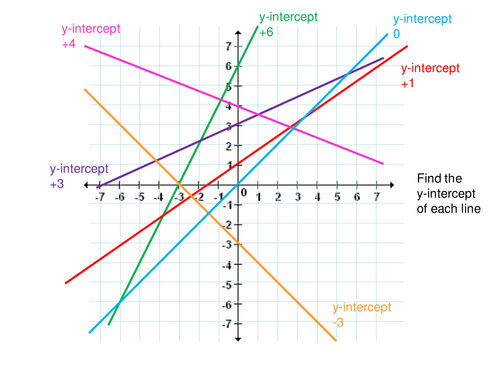Straight Line Graphs
4 years ago
mrkennedy
Save
Edit
Host a game
Live GameLive
Homework
Solo Practice
Practice15 QuestionsShow answers
• Question 1
300 secondsQ. What is the gradient of the line
y = 2x - 3?
2
-3
3
2x
• Question 2
300 seconds
Q. Which of these lines would be the steepest?
y = 2x + 9
y = 7x + 3
y = 5x - 3
y = 7
• Question 3
300 secondsQ. Which of these lines will intercept the y-axis at 5?
y = 5x
y = x - 5
5y = 5x + 5
y = x + 5
• Question 4
300 secondsQ. What is the y-intercept of the line
y = 4x - 6?
4
4x
6
-6
• Question 5
300 secondsQ. Which of these lines has a negative gradient?
The orange one
The red one
The blue one
The green one
• Question 6
300 secondsQ. What is the gradient of this line?
2
½
-3
• Question 7
300 secondsQ. What is the y-intercept of this line?
2
3
-3y
-3
• Question 8
300 secondsQ. What is the equation of this line?
(in the form y = mx + c)
y = -3x + 2
y = 2x - 3
y = 2x + 3
y = -2x - 3
• Question 9
300 secondsQ. Which of these lines is
y = 5?
The orange one
The red one
The blue one
The green one
• Question 10
300 secondsQ. Which of these lines has a gradient of ½?
The orange one
The red one
The blue one
The green one
• Question 11
300 secondsQ. What rule connects the and y values from this table?
y = 2x + 5
y = 4x + 1
y = 3x + 3
y = x + 7
• Question 12
300 secondsQ. What rule connects the and y values from this table?
y = x + 8
y = 2x + 3
y = 3x - 2
y = 4x - 7
• Question 13
300 secondsQ. Which of these
co-ordinates lie on the line
y = 3x - 8?
(5, 8)
(0, 8)
(2, -2)
(1, 5)
• Question 14
300 secondsQ. Which of these lines passes through the point (3, 7)?
y = 2x + 4
y = x + 5
y = x + 10
y = 3x - 2
• Question 15
300 secondsQ. The point A on the line
y = 3x - 7
has an x co-ordinate of 4.
What is the y co-ordinate of A?